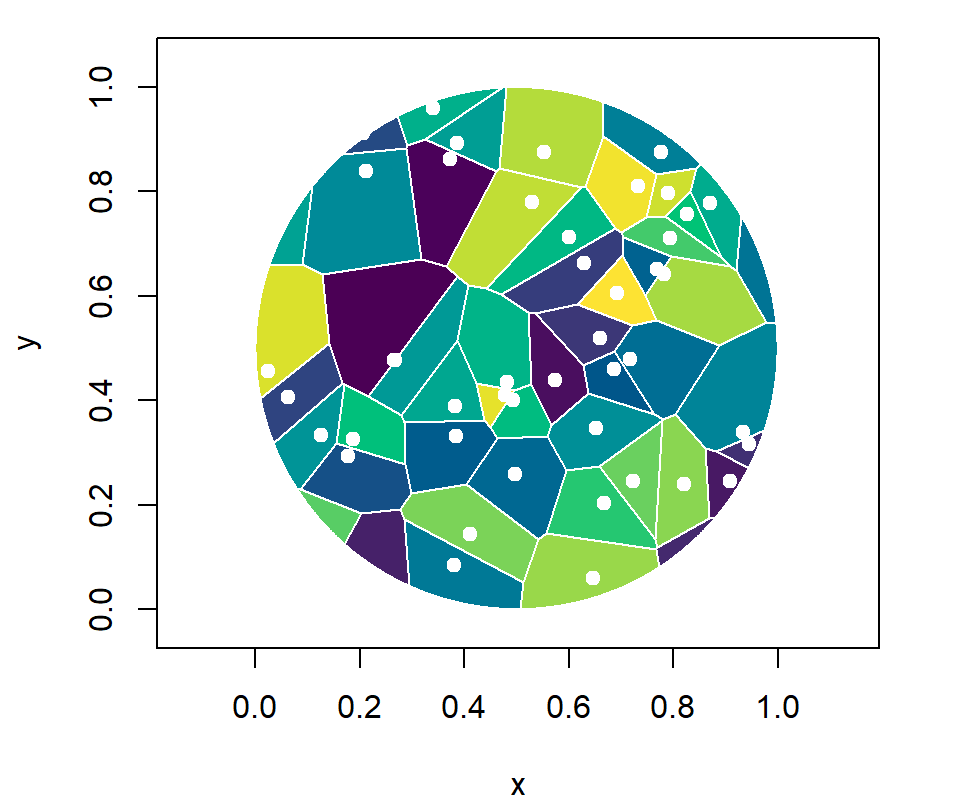# Voronoi diagrams in R with deldir

Rolf Turner

## Voronoi diagram with `deldir`

A Voronoi diagram (or Thiessen polygons) can be created in base R with the functions provided by `deldir` package. Given two vectors you will need to calculate the Delaunay triangulation with the `deldir` function and the Dirichlet (Voronoi) tessellation with the `tile.list` function. Then, you can plot the resulting object with the `plot.tile.list` S3 method.

``````# install.packages("deldir")
library(deldir)

# Data
set.seed(1)
x <- runif(50)
y <- runif(50)

# Calculate Voronoi Tesselation and tiles
tesselation <- deldir(x, y)
tiles <- tile.list(tesselation)

plot(tiles, pch = 19)``````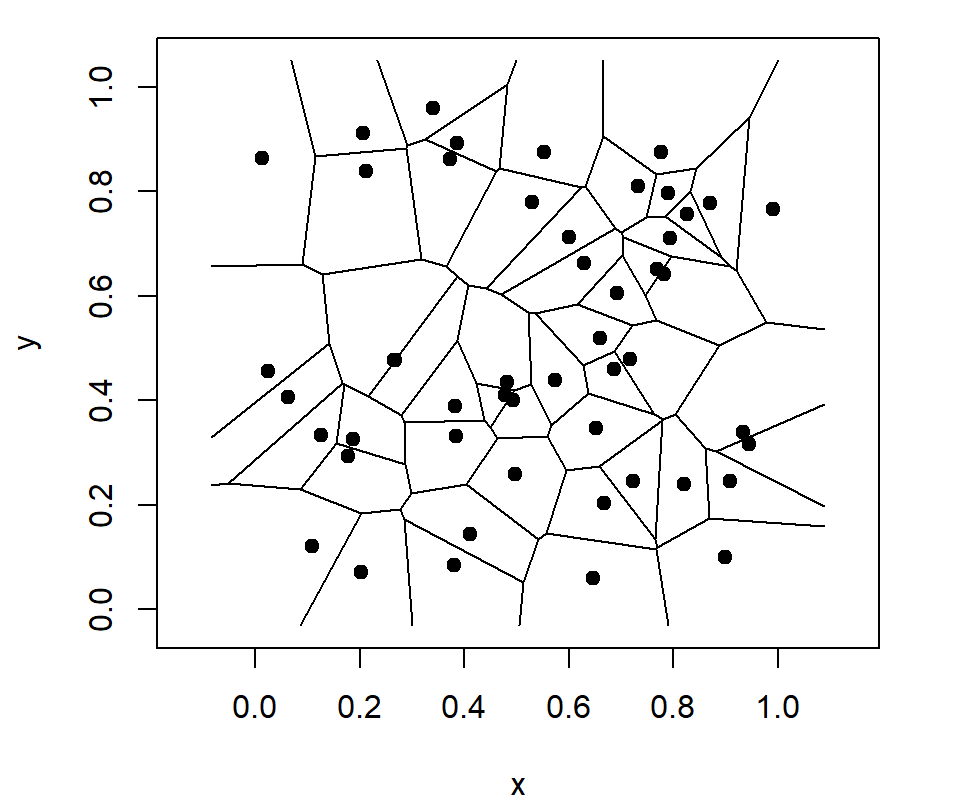If you want you can add numbers instead of the default points, setting `number = TRUE`.

``````# install.packages("deldir")
library(deldir)

# Data
set.seed(1)
x <- runif(50)
y <- runif(50)

# Calculate Voronoi Tesselation and tiles
tesselation <- deldir(x, y)
tiles <- tile.list(tesselation)

plot(tiles, pch = 19,
number = TRUE)``````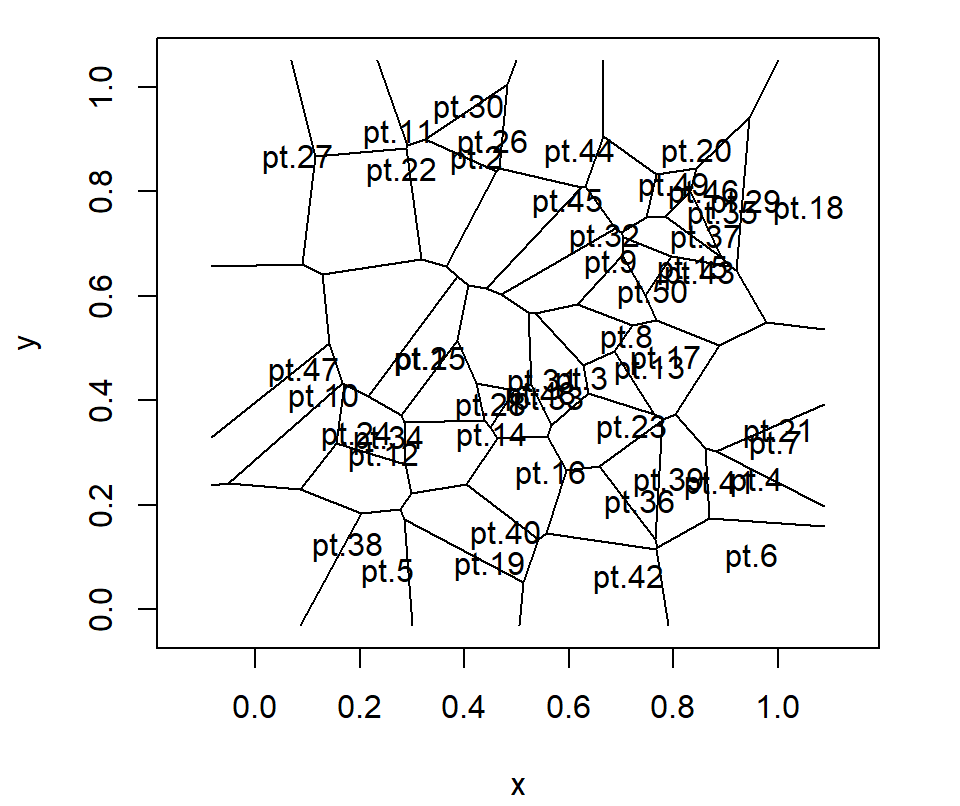In addition, it is possible to remove the points setting the argument `showpoints` to `FALSE`.

``````# install.packages("deldir")
library(deldir)

# Data
set.seed(1)
x <- runif(50)
y <- runif(50)

# Calculate Voronoi Tesselation and tiles
tesselation <- deldir(x, y)
tiles <- tile.list(tesselation)

plot(tiles,
showpoints = FALSE)``````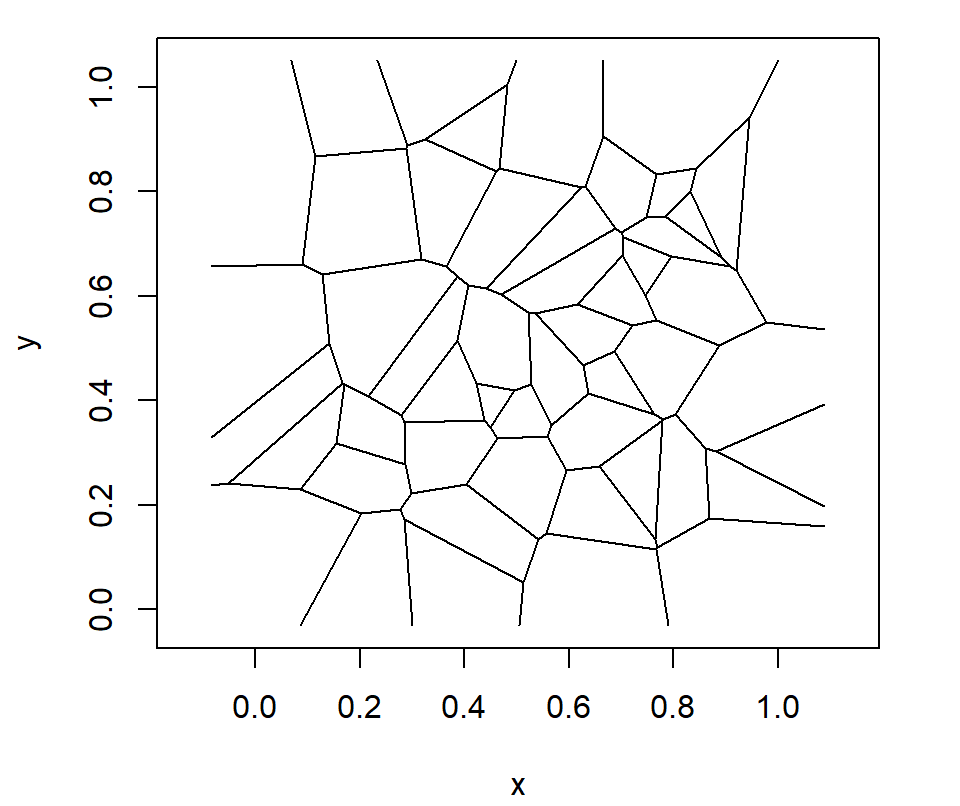The edges of the tiles can be closed with a rectangle setting `close = TRUE`.

``````# install.packages("deldir")
library(deldir)

# Data
set.seed(1)
x <- runif(50)
y <- runif(50)

# Calculate Voronoi Tesselation and tiles
tesselation <- deldir(x, y)
tiles <- tile.list(tesselation)

plot(tiles,
close = TRUE)``````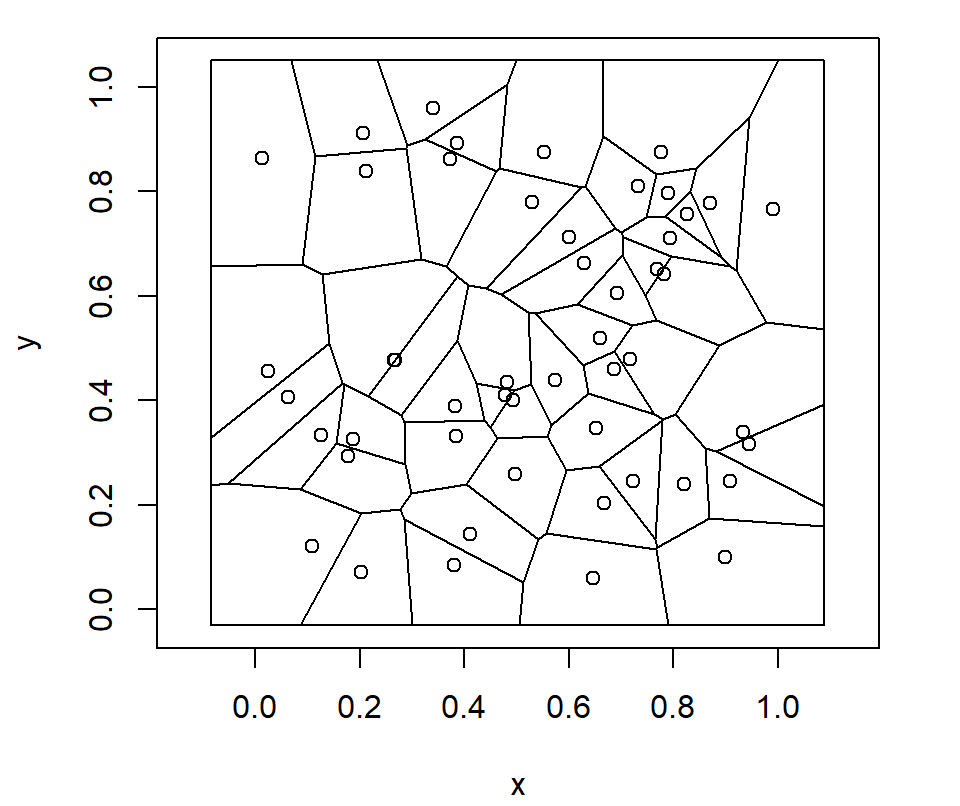## Fill and border colors

You can pass a color palette to the `fillcol` argument of the plotting function to add colors to the tiles. Note that you can also change the color or the points with `col.pts` or the color of the numbers with `col.num`. The latter in case `number = TRUE`.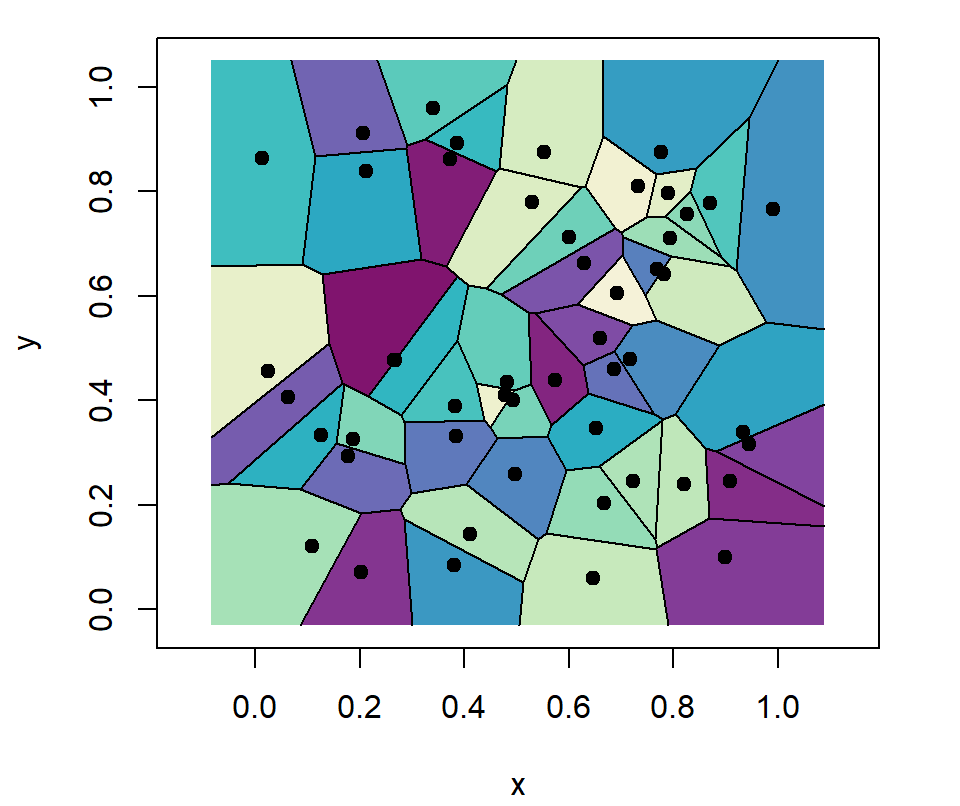``````# install.packages("deldir")
library(deldir)

# Data
set.seed(1)
x <- runif(50)
y <- runif(50)

# Calculate Voronoi Tesselation and tiles
tesselation <- deldir(x, y)
tiles <- tile.list(tesselation)

plot(tiles, pch = 19,
fillcol = hcl.colors(50, "Purple-Yellow"))``````

The `border` argument allows changing the color of the border of the tiles, as shown in the example below.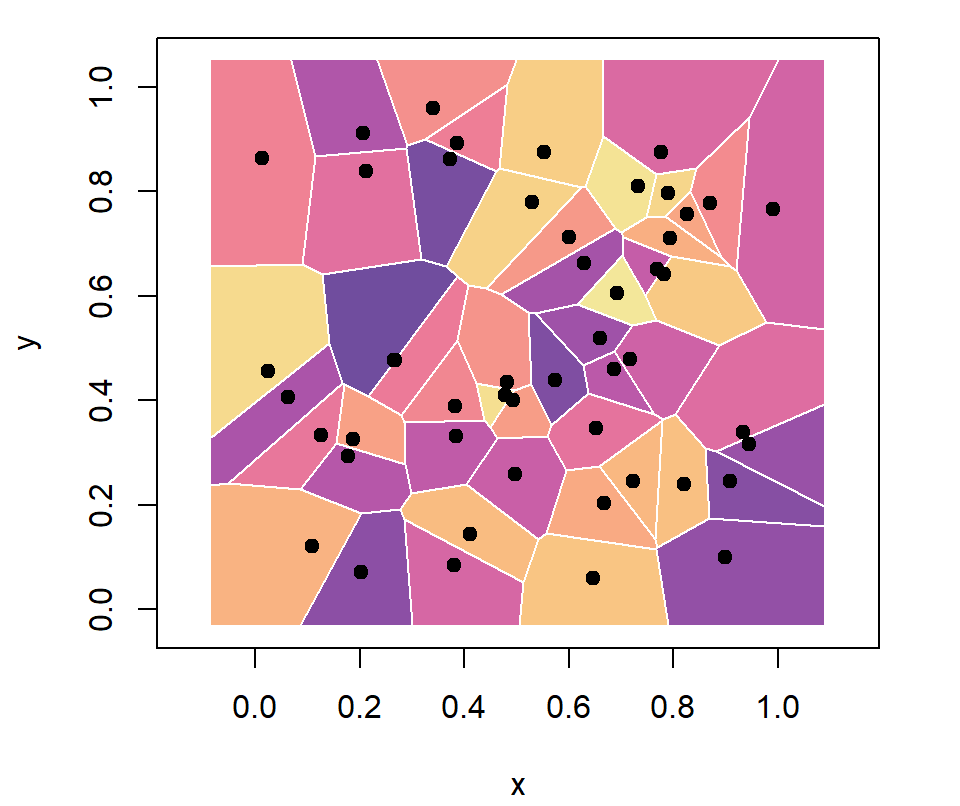``````# install.packages("deldir")
library(deldir)

# Data
set.seed(1)
x <- runif(50)
y <- runif(50)

# Calculate Voronoi Tesselation and tiles
tesselation <- deldir(x, y)
tiles <- tile.list(tesselation)

plot(tiles, pch = 19,
border = "white",
fillcol = hcl.colors(50, "Sunset"))``````

## Clip shape

The function also provides a clipping method with `clipp`. The argument needs a list with the components `x` and `y` giving the coordinates of the polygon to which the tessellation should be clipped. In the following example we are clipping it to a circle.

``````# install.packages("deldir")
library(deldir)

# Data
set.seed(1)
x <- runif(50)
y <- runif(50)

# Calculate Voronoi Tesselation and tiles
tesselation <- deldir(x, y)
tiles <- tile.list(tesselation)

# Circle
s <- seq(0, 2 * pi, length.out = 3000)
circle <- list(x = 0.5 * (1 + cos(s)),
y = 0.5 * (1 + sin(s)))

plot(tiles, pch = 19,
col.pts = "white",
border = "white",
fillcol = hcl.colors(50, "viridis"),
clipp = circle)``````